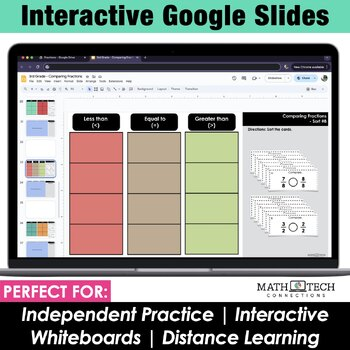# 3rd Grade Fractions Math Centers 3rd Grade Interactive Math Notebook Math Sorts3rd
Subjects
Standards
Resource Type
Formats Included
• Zip
Pages
24 + Organization

#### Also included in

1. These math centers are engaging activities that encourage math talk. Students explain how they sort their cards and build strong math concepts as they create cards of their own.What is Included?- 81 sorts that review third grade math standards. - Math Sorts Binder - Pages that list all math sorts,
\$25.00
\$38.50
Save \$13.50
2. This third grade math bundle includes 5 AMAZING MATH RESOURCES! Included: Math Tri-Folds, Math Sorts, Math Morning Work, Math Homework, and Math Interactive Notebook! The resources included are flexible and may be used for morning work, whole group practice, guided math practice, independent practic
\$92.80
\$176.36
Save \$83.56
3. This 3rd grade math bundle includes no-prep math sorting activities that can be used as partner math centers, math warm ups, or math exit tickets. This 3rd grade math practice bundle includes both PRINT and DIGITAL format. - The printable format includes 81 cut-and-past activities- The paperless for
\$40.00
\$80.00
Save \$40.00

### Description

These math centers are engaging, interactive activities that encourage math talk. Students explain how they sort their cards and build strong math concepts as they create cards of their own.

INCLUDED:

- 12 math sorts (2 versions)

- Math Sorts Binder - Pages that list all math sorts, standard, topic, and small group lesson plan page for notes

- Blank PowerPoint Template - Just add text boxes to the template and create your own sorts!

- Student Notebook Dividers - Students keep their math sorts notebook organized with these dividers. Includes learning goals! The dividers are now editable.

- Student Directions for Day 1 and Day 2 Activities

Ways to Use this Resource

- Add to a math center for students to complete independently. Have students discuss with a partner how they organized their cards.

- Send home as a homework activity

- Quick assessment

* 3rd Grade Math Standards Covered *

- 3.NF.1:

Sort 1: Equal parts, unequal parts

Sort 2: 3 equal parts, 4 equal parts, 6 equal parts

Sort 4: three fourths, two sixths, or five eights shaded

Sort 5: one half, one third, or one fourth shaded

- 3.NF.2

Sort 6: Fractions on a number line: one half, one third, one fourth

Sort 7: Improper fractions (greater than 1) and mixed numbers on a number line

- 3.NF.3

Sort 8: Fractions less than 1/2, equal to 1/2, or greater than 1/2

Sort 9: Fractions equal to one whole and not equal to one whole

Sort 10: Fractions equal to one whole, two wholes, or three wholes

Sort 11: True or False comparisons

Sort 12: Compare fractions (greater than, less than, or equal to)

There are 2 versions for each sort, so you can differentiate as needed. Version 1 is more challenging, because the category names are not given. Version 2 gives the category names.

MATH SORTS Available Now:

----------------------------------------------

PURCHASE THE BUNDLE AND SAVE!! - - - -> Click Here - 80 sorts!!

----------------------------------------------

- Set 1: Number & Operations in Base Ten - 14 sorts

- Set 2: Geometry - 13 sorts

- Set 3: Fractions - 12 sorts

- Set 4: Measurement & Data - 16 sorts

- Set 5: Operations & Algebraic Thinking - 25 sorts

------------------------------------

------------------------------------

You may also be interested in....

Total Pages
24 + Organization
Included
Teaching Duration
N/A
Report this Resource to TpT
Reported resources will be reviewed by our team. Report this resource to let us know if this resource violates TpT’s content guidelines.

### Standards

to see state-specific standards (only available in the US).
Compare two fractions with the same numerator or the same denominator by reasoning about their size. Recognize that comparisons are valid only when the two fractions refer to the same whole. Record the results of comparisons with the symbols >, =, or <, and justify the conclusions, e.g., by using a visual fraction model.
Express whole numbers as fractions, and recognize fractions that are equivalent to whole numbers. Examples: Express 3 in the form 3 = 3/1; recognize that 6/1 = 6; locate 4/4 and 1 at the same point of a number line diagram.
Recognize and generate simple equivalent fractions, (e.g., 1/2 = 2/4, 4/6 = 2/3). Explain why the fractions are equivalent, e.g., by using a visual fraction model.
Understand two fractions as equivalent (equal) if they are the same size, or the same point on a number line.
Explain equivalence of fractions in special cases, and compare fractions by reasoning about their size.